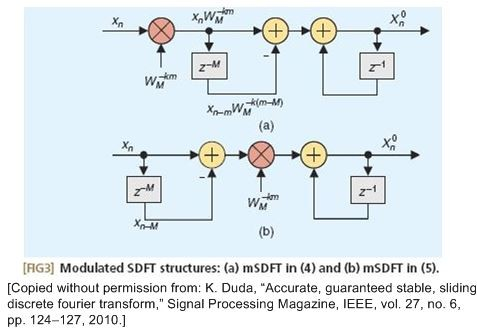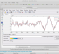(So far, 86 people got it right out of 205 for a success rate of 41%)

Linear time-invariant (LTI) systems have a useful commutative property where their sequential order can be rearranged with no change in their final outputs.This situation, described in almost every DSP textbook, is shown in Figure 1 where two different LTI systems are configured in series. Swapping the order of two cascaded LTI systems does not alter the final output. That is, y1(n) is always equal to y2(n).The textbooks don't come right out and say it but they strongly imply that swapping the order of the two systems in Figure 1 is not permitted if either System #1 or System #2 is not LTI.

Now consider the cascaded system, that I encountered recently, shown in Figure 2(a). Variable n is the integer time index, k is a real-valued frequency variable, and N is a positive integer. The multiplication in Stage #2 is not LTI because it is not time-invariant.Quiz Question: Under what condition can we swap the order of the stages in Figure 2(a) to create Figure 2(b) such that y1(n) = y2(n)

Pick one:
When k is negative
When k is an integer
When 2πk(n-1)/N is aperiodic
None of the above

[ - ]
Comment by June 12, 2020The answer appears (to me) to be stated a little too loosely.

kn/N is rational
<=>  k/N is rational (since n can be any integer)
<=>  k is rational (since N is a positive integer)

But a rational k is not the same as an integer k.

For example, taking N = 100 and k = 1/2, k/N = (1/2)/(100) = 1/200 which is clearly rational (as it will be nk/N, since this latter quantity is simply an integer multiple of the former), but the following two functions are not equal for all n:

cos[2*pi*(1/2)*(n/100)] =/= cos[2*pi*(1/2)(n-100)/100] = cos[2*pi*(1/2)(n/100-1)] = cos[2*pi*(1/2)(n/100)-pi] = -cos[2*pi*(1/2)(n/100)]

I suspect this counterexample isn't really needed since I'm sure we all agree that k must be an integer, however the key point being that this is not the same as nk/N (or k) being rational.

[ - ]
Comment by June 12, 2020Hi Michael Collins. Oh shoot, you are correct. In my Quiz Questions I like to make the potential answers a little "tricky". In not wanting to have the simple, and always correct, "When k is an integer" be one of the possible answers I converted that answer to "When nk/N is rational", which as you say is not always correct. Good catch Michael!!

The origin of this Quiz Question:

I was recently studying the following diagrams within a spectrum analysis paper where variable m is the time-domain index.The paper's author swapped the multiplier and comb in the top network to create the bottom network. I accepted that swapping without much thought. Then, literally, I woke up in the middle of the night and thought, "You can't do that! You can't swap the multiplier and comb." But after a little study I realized you could perform that swapping if frequency variable k was an integer. And that's when a "Quiz Question" occurred to me.

[ - ]
Comment by June 12, 2020Thanks, Rick. My post was as much to test my own understanding than aimed at correcting you -- there's enough such people lying in wait on the wild wild web already!

I enjoy these quizzes as they test one's knowledge of DSP fundamentals, which can be intricate and subtle, often with many possible angles to the solution. After many years as an engineer, I'm still learning interesting things about the plain old 'simple' DFT.

[ - ]
Comment by June 12, 2020Hi Michael. It was a pleasure to read your sensible words. Regarding your comment that there still are interesting things to learn about the plain old 'simple' DFT, Rocky Balboa would reply with "That is very true."

[ - ]
Comment by June 12, 2020Hey Rick,

What is the new score?

Dirk

[ - ]
Comment by June 12, 2020Hi Dirk. My my. I haven't heard from you in many years. I assumed you either won the Lottery and were living on a sailboat off the coast of a Greek island or you were abducted by an alien Mother Ship. I hope you're doing well. In any case, what score are you referring to?

[ - ]
Comment by June 12, 2020Hey Rick,

I wish one of those options was true 😀. Still doing DSP.

I meant the number of people who got the right answer. It looks like it was updated.

Dirk

[ - ]
Comment by June 13, 2020Hi Dirk.

As of June 13, the "score" is 27 people got it right out of 53 for a success rate of 50%

[ - ]
Comment by June 12, 2020Yes, k an integer is not the solution?

[ - ]
Comment by June 12, 2020Hi omersayli. You should consider modeling the two cascaded networks with software, setting k to a non-integer value and then set k to an integer value, to test your statement.

[ - ]
Comment by June 23, 2020Hi Rick,

I made Python-Jupyter notebook for making a random signal and seeing the outputs of Fig2a and Fİg2b with changing k and N. It was a good exercise for me to use Google colab to share a notebook also.

(select fork this project, run Quiz11.ipynb)

Colab link; (select google colab to open, run each cell from top to bottom)

[ - ]
Comment by June 13, 2020The condition nk/N must be rational does not imply that k is an integer.k could be for example simply 2/3 then also nk/N is rational.But this will not work as 2*pi*k will not be a multiple of 2*pi..So correct choice must be none of the above

[ - ]
Comment by June 13, 2020Hello Mannai_Murali. You are correct. Please see the above first June 12 Comment by Michael Collins and my June 12 reply to his comment.

[ - ]
Comment by June 14, 2020I've frequently used precisely this question as a bonus question on final exams. Other similar bonus questions have been:

1) give examples of two systems that don't commute because a) exactly one is not TI b) exactly one is nonlinear

2) is time reversal y_n = x_{-n}  TI ? (explain)

3) what is the effect on the spectrum of a signal of inverting every other sample (i.e., x_n -> -x_n for odd n)?

Y(J)S

[ - ]
Comment by June 14, 2020Hi Y(J)S.

I'll stick my neck out regarding your question# 2 and say, "For both "flip" (left/right) time-reversal and circular time-reversal the answer is No."